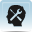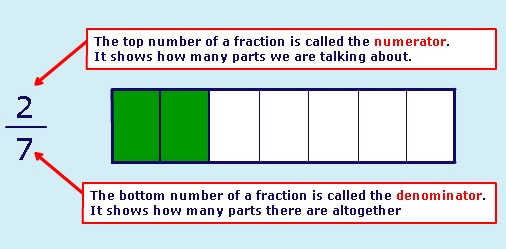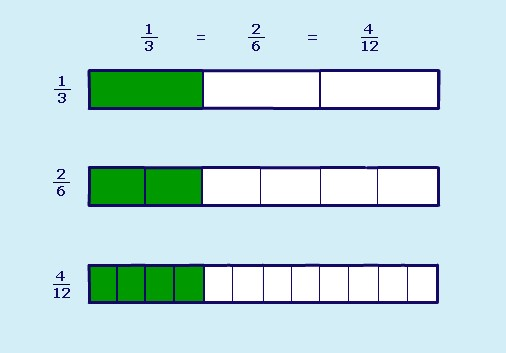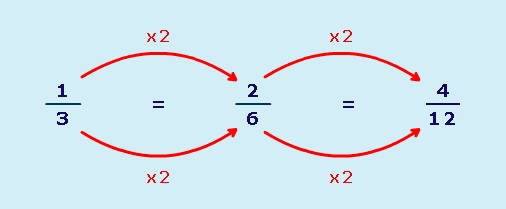# Resource 1: FractionsBackground information / subject knowledge for teacher

## What is a fraction?

A fraction is a part of a whole. There are two numbers to every fraction:## Equivalent fractions

Equivalent fractions are fractions that look different but show exactly the same amount:You can make equivalent fractions by multiplying or dividing the numerator and denominator by the same number:Original source: http://www.bbc.co.uk/ schools (Accessed 2008)

3. Using group work to explain equivalent fractions

Resource 2: Practical fractions